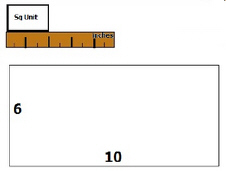3.MD.7.B.-Test1B Multiply side lengths to find areas of rectangles
 Name:    3.MD.7.B.-Test1B Multiply side lengths to find areas of rectangles

Multiple Choice
Identify the choice that best completes the statement or answers the question.

1.

What is the area of a rectangle with a length of 20 cm and width of 9 cm?
 a. 180 sq cm c. 11 sq cm b. 160 sq cm d. 29 sq cm

2.

What is the area of a rectangle that has a length of 13 cm and a width of 8 cm?
 a. 5 sq cm c. 21 sq cm b. 94 sq cm d. 104 sq cm

3.

Maria needed some blocks to build a fort for her doll.  What is the area of a rectangular fort she built that has a length of 15 in and a width of  5 in?
 a. 50 sq in c. 5 sq in b. 75 sq in d. 15 sq in

4.

Eddie had some Legos and he wanted to build a base to set his trophy on.  What is the area of the base if it has a length of 12 cm and a width of 4 cm?
 a. 61 sq cm c. 58 sq cm b. 15 sq cm d. 48 sq cm

5.

What is the area of a rectangle that has a length of 11 km and a width of 9 km?
 a. 90 sq km c. 2 sq km b. 99 sq km d. 20 sq km

6.

Patty was creating a grid for a game. She measured the squares she would put on the game board.  The board is 6 in by 10 in.  How many square units will she need?  Use the diagram to figure it out.  Be sure to check out the measurement data in the diagram.a. 18 c. 15 b. 9 d. 9

7.

What is the area of a rectangle that has a length of 5 km and a width of 2 km?
 a. 7 sq km c. 10 sq km b. 3 sq km d. 10 sq km

8.

What is the area of a rectangle that has a length of 7 m and a width of 6 m?
 a. 13 sq m c. 1 sq m b. 42 sq m d. 43 sq m

9.

What is the area of a rectangle that has a length of 16 in and a width of 6 in?
 a. 10 sq in c. 22 sq in b. 66 sq in d. 96 sq in

10.

What is the area of a rectangle that has a length of 6 cm and a width of 9 cm?
 a. 54 sq cm c. 53 sq cm b. 3 sq cm d. 15 sq cm